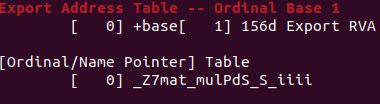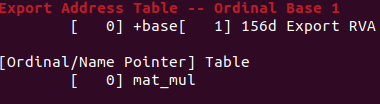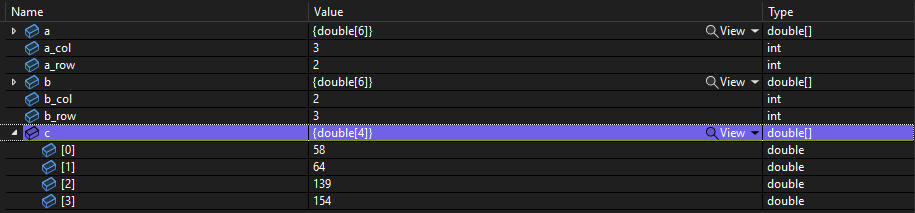Numerical algorithms are often developed in Python due to ease of prototyping, and because many of the libraries that do the heavy lifting are written in fast, compiled languages, so performance is not usually an issue. However, there are often cases where you don’t want to use Python. For instance, you may not want to deploy an application containing sensitive IP written in a scripting language (even in the form of Python byte code) on a client PC. As an algorithm developer, in many such cases you will be working with another team to implement the algorithm into a GUI Windows application, often written in something like C#. For such cases, it is useful to know how to cross-compile C++ code from Linux to target a DLL for Windows deployment, and how to interoperate between C++ and C#.

We will go through a complete example of cross-compiling a very simple C++ program using MinGW to build a DLL and show how to call that DLL from a C# Windows application. First: our “numerical algorithm”, matrix multiplication, where the matrix is a std::vector of std::vector<double>’s:

#include <vector>

typedef std::vector<std::vector<double>> mat;

mat mmul(mat a, mat b){
mat res;
int a_row = a.size();
int a_col = a.size();
int b_col = b.size();
double smr = 0.;
for(int i = 0; i < a_row; i++){
std::vector<double> res_i;
res.push_back(res_i);
for(int j = 0; j < b_col; j++){
for(int k = 0; k < a_col; k++){
smr += a[i][k]*b[k][j];
}
res[i].push_back(smr);
smr = 0.;
}
}
return res;
}



Now, if we want to use this method directly from a C# application, we need a wrapper method because C# is not going to be able to interoperate directly with C++ types, so we will need to pass arguments into our wrapper as flattened (1D) C-style arrays.

#ifdef _WIN32
extern "C" __declspec(dllexport)
#endif
void mat_mul(double a[], double b[], double c[], int a_row, int a_col,
int b_row, int b_col){
mat a_mat;
mat b_mat;
std::vector<double> a_i;
std::vector<double> b_i;
int cntr = 0;
for(int i = 0; i < a_row; i++){
for(int j = 0; j < a_col; j++){
a_i.push_back(a[cntr]);
cntr++;
}
a_mat.push_back(a_i);
a_i.clear();
}
cntr = 0;
for(int i = 0; i < b_row; i++){
for(int j = 0; j < b_col; j++){
b_i.push_back(b[cntr]);
cntr++;
}
b_mat.push_back(b_i);
b_i.clear();
}
mat res = mmul(a_mat, b_mat);
cntr = 0;
for(int i = 0; i < a_row; i++){
for(int j = 0; j < b_col; j++){
c[cntr] = res[i][j];
++cntr;
}
}
return;
}


A couple of notes with respect to the wrapper function. _WIN_32 is a macro defined in toolchains targeting Windows, for instance when using the MSVC compiler or the MinGW compiler; it will not be defined if you compile with the Linux system C++ compiler, for instance for debugging. The extern "C" indicates that we want to use C style linkage for the function so that we know the function name. Without this, the function name will be mangled in a non-standard, implementation specific way to account for features of the C++ language like function overloading. For instance, if I remove the extern "C" and objdump -x the relevant part of the MinGW compiled DLL via objdump -x mat_mul.dll | grep "Export Address Table -- Ordinal Base 1" -A5 I seeHowever, compiling with extern "C" yieldsso we can reliably use this function based on the name given in the source code. __declspec(dllexport) is a Windows specific attribute telling the compiler that you want this function exposed in the DLL. Finally, note that I am passing in an array c, which will be our result array.

With these two functions and the MinGW compiler, we can build our DLL targeting an x86-64 Windows machine via x86_64-w64-mingw32-g++ -Wall mat_mul.cpp -shared -static -o mat_mul.dll. Note the -shared flag, telling the compiler that we are building a DLL, and the -static flag, telling the linker that we do not want to link against dynamic libraries. This will yield a relatively large DLL, a few Mb in this case, due to the static linking, but this simplifies deployment.

With the DLL in hand (make sure it is placed in a directory that is in the Windows machine’s PATH environment variable), we can write a simple C# application to call the DLL. First, we create a class just to declare our external method.

using System.Runtime.InteropServices;

class cpp_function
{
[DllImport("mat_mul.dll", EntryPoint = "mat_mul")]
public static extern void mat_mul(double[] a, double[] b, double[] c, int a_row,
int a_col, int b_row, int b_col);
}


And we also define a class with our main method, calling our external method.

class cpp_call
{
static void Main()
{
double[] a = new double[] { 1, 2, 3, 4, 5, 6 };
double[] b = new double[] { 7, 8, 9, 10, 11, 12 };
double[] c = new double[] { 0, 0, 0, 0 };
int a_row = 2;
int a_col = 3;
int b_row = 3;
int b_col = 2;
cpp_function.mat_mul(a, b, c, a_row, a_col, b_row, b_col);
}
}


Here, we are passing in our target arrays in row-major order, so we are multiplying a

$\begin{bmatrix} 1 & 2 & 3 \\ 4 & 5 & 6 \\ \end{bmatrix}$

and b

$\begin{bmatrix} 7 & 8 \\ 9 & 10 \\ 11 & 12 \\ \end{bmatrix}$

with the resulting matrix placed in our zero initialized array c. The result should be

$\begin{bmatrix} 58 & 64 \\ 139 & 164 \\ \end{bmatrix}$

which will be

$\begin{bmatrix} 58 & 64 & 139 & 164 \\ \end{bmatrix}$

when flattened.

With these classes in our C# source file within Visual Studio, we can compile our application simply by pressing Build. So that we can see the array c containing the results from our matrix multiplication, I placed a breakpoint at the end of Main.Note that c contains the expected results from our matrix multiplication, so we have successfully called our C++ method, with the resultant data available in our C# application.